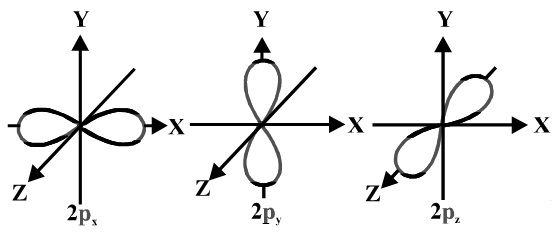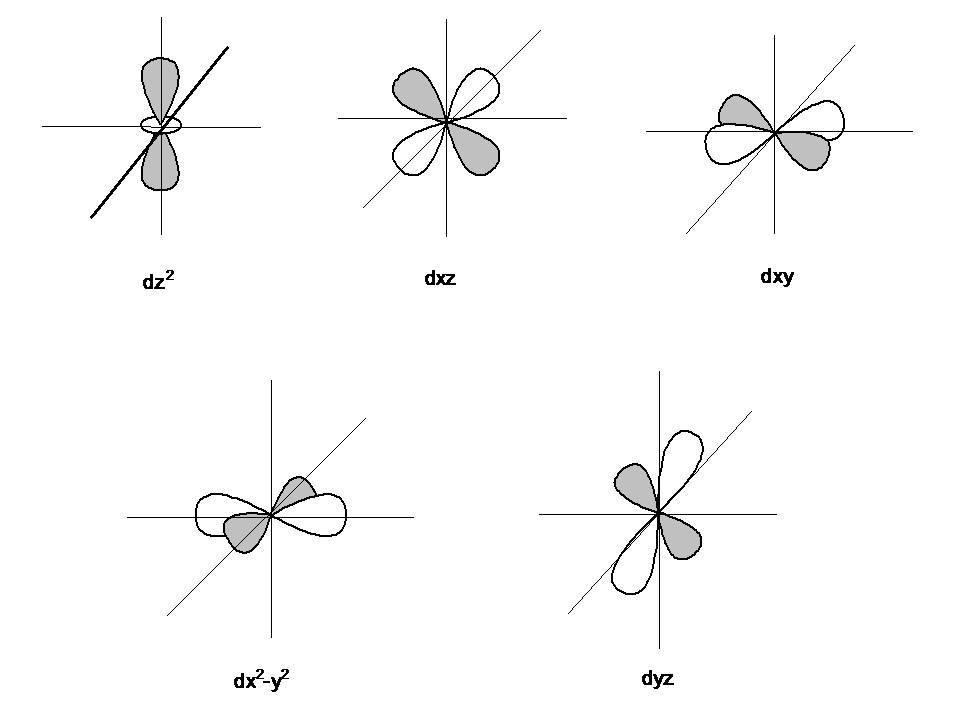# How do you draw s,p,d,f orbitals?

Jan 29, 2014

For an $s$ orbital, draw a circle; for a $p$ orbital, draw a figure eight; for a $d$ orbital, draw a four-leafed clover; for an $f$ orbital, see below.

#### Explanation:

An $s$ orbital is a sphere. In two dimensions, we draw it as a circle.

A $p$ orbital consists of two lobes of electron density on either side of the nucleus.

We usually draw $p$ orbitals as figure eights, but we should remember $p$ orbitals are really much fatter than in our usual drawings.Four of the five $d$ orbitals consist of four lobes pointed towards the corners of a square, like a four-leaf clover. The fifth orbital looks like a $p$ orbital with a doughnut around its middle.We usually draw $d$ orbitals as skinny teardrops pointing in various directions, but we should remember that the lobes are really much fatter than in the drawing.

The seven $f$ orbitals are even more complex shapes. The ones we see in diagrams are linear combinations of the ${m}_{l}$ = -3 to +3 orbitals. Here is one common set of combinations.If you absolutely have to draw them, you can draw the shapes as below.• One orbital looks like a $p$ orbital with two doughnuts around its middle.
• Two orbitals have eight lobes pointing towards the corners of a cube.
• Four orbitals have six lobes oriented in various planes (easiest to draw).